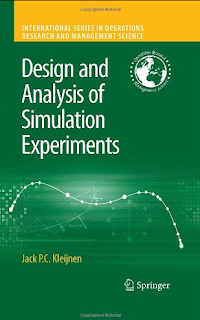## Social Items

This is an advanced expository book on statistical methods for the Design and Analysis of Simulation Experiments (DASE). Though the book focuses on DASE for discrete-event simulation (such as queuing and inventory simulations), it also discusses DASE for deterministic simulation (such as engineering and physics simulations). The text presents both classic and modern statistical designs. Classic designs (e.g., fractional factorials) assume only a few factors with a few values per factor. The resulting input/output data of the simulation experiment are analyzed through low-order polynomials, which are linear regression (meta)models. Modern designs allow many more factors, possible with many values per factor. These designs include group screening (e.g., Sequential Bifurcation, SB) and space filling designs (e.g., Latin Hypercube Sampling, LHS). The data resulting from these modern designs may be analyzed through low-order polynomials for group screening and various metamodel types (e.g., Kriging) for LHS.## Design and Analysis of Simulation Experiments (Jack P.C. Kleijnen) - KLIKCUY.COM

This is an advanced expository book on statistical methods for the Design and Analysis of Simulation Experiments (DASE). Though the book focuses on DASE for discrete-event simulation (such as queuing and inventory simulations), it also discusses DASE for deterministic simulation (such as engineering and physics simulations). The text presents both classic and modern statistical designs. Classic designs (e.g., fractional factorials) assume only a few factors with a few values per factor. The resulting input/output data of the simulation experiment are analyzed through low-order polynomials, which are linear regression (meta)models. Modern designs allow many more factors, possible with many values per factor. These designs include group screening (e.g., Sequential Bifurcation, SB) and space filling designs (e.g., Latin Hypercube Sampling, LHS). The data resulting from these modern designs may be analyzed through low-order polynomials for group screening and various metamodel types (e.g., Kriging) for LHS.
Load Comments

Subscribe Our Newsletter